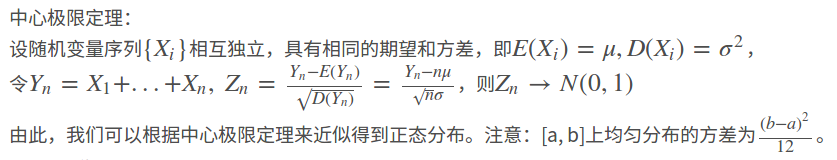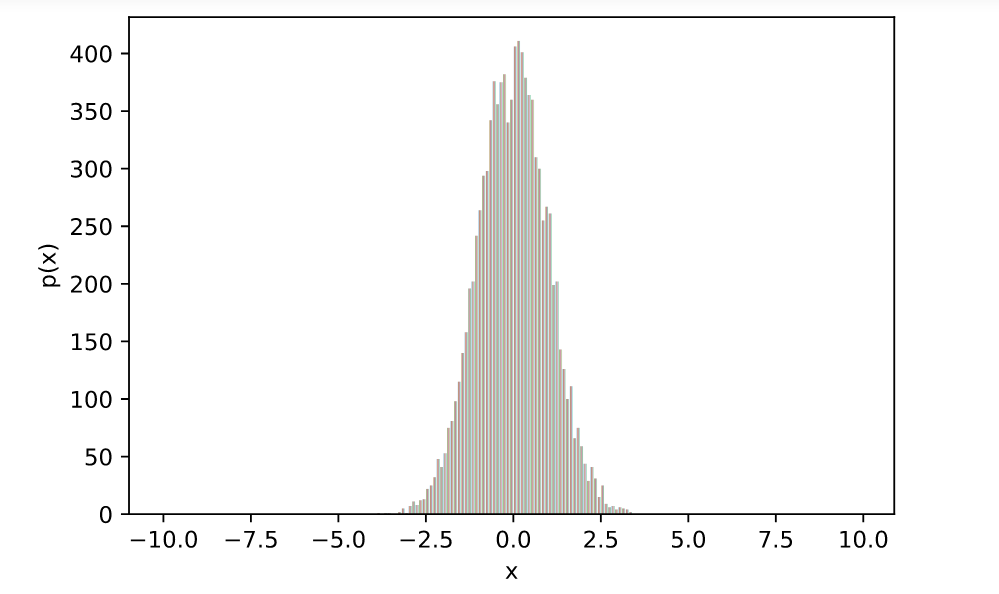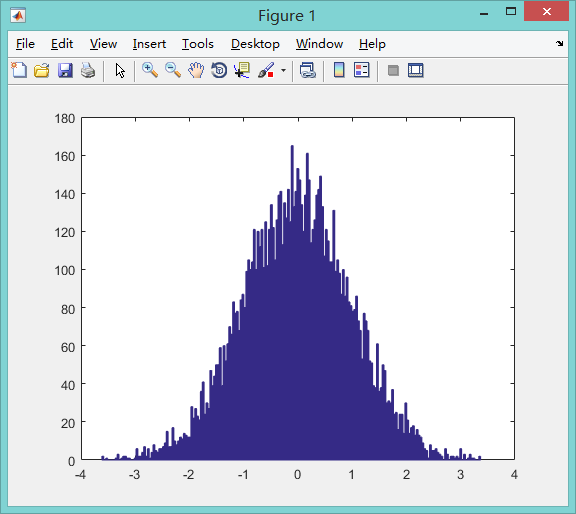• 在本示例中，选择a=0，b=1，这个均匀分布的方差等于（1/12）。 #定义高斯采样函数 def gauss_rand(): n = 100; #均匀产生100个0~1之间的随机数 u = np.random.uniform(0,1,n) r = (sum(u) - n * 0.5) / np...在本示例中，选择a=0，b=1，这个均匀分布的方差等于（1/12）。
#定义高斯采样函数
def gauss_rand():
n = 100;
#均匀产生100个0~1之间的随机数
u = np.random.uniform(0,1,n)
r = (sum(u) - n * 0.5) / np.sqrt(n/ 12);
return r

#使用高斯采样函数进行采样
num = 10000;
for i in range(num):
x[i] = gauss_rand()

#绘制直方图，显示随机变量的概率分布
bins = np.arange(-10, 10, 0.1)
plt.hist(x, bins=bins)
plt.show()对应的matlab代码：利用均匀分布和中心极限定理产生正态分布（高斯分布）


展开全文• 　x为均匀分布的变量，y为另一分布的变量，利用其累计函数作为中间关系，来完成映射。那么问题又来了，为什么可以这样呢？如何来理解这一问题呢？ 来两张图感性认识下：   前者是0到100的均匀分布累计函数，...
　　首先交代下背景，课题需要产生服从高斯分布的随机变量，这个要求对于python，Matlab而言，也就是一个函数调用的事（其实C++的库里面也有，无奈之前不知道，(⊙o⊙)…），假如不调用，我们自己应当如何实现呢？或者再延伸下，如果我们需要产生任意分布，这下没函数调用了吧，那么我们应该怎么办呢？这就是一个比较有意思的问题了......
首先来看看我们有啥可以用的，一般而言我们是可以获取随机数的（其实这个随机数也是伪随机数，可以通过线性同余法来产生，这是后话了），我们可以把这个数看做服从均匀分布的随机变量，那么如何将一个均匀分布的变量映射到另一个分布的变量呢？
答案如下：x为均匀分布的变量，y为另一分布的变量，利用其累计函数作为中间关系，来完成映射。那么问题又来了，为什么可以这样呢？如何来理解这一问题呢？
来两张图感性认识下：前者是0到100的均匀分布累计函数，后者是N(50,25)的累计函数，可以发现：
1.二者均为递增函数(可以看做严格单调，尽管不明显)
2.函数值范围为[0,1]
映射的原理就是在由均匀分布变量的值找到其累计函数值，对应到图二中相同函数值所对应变量值，由上面两幅图可以发现，图一中几乎所有值都对应到了图二中的40~60中，这在直观上和正态分布的效果是相符的。
接下来就看看如何来程序实现，

//---------------------GaussianDistribution.h------------------------
#pragma once
//#include "Interface.h"
#define SP 1000//设置精确度，取整个正态分布的区间分为SP等份
namespace RAN
{
class GaussianDistribution 	{
public:
GaussianDistribution(void);
~GaussianDistribution(void);
double u,d,step;
double mp[SP],mf[SP];//mp为正态分布区间每等份所对应的概率密度，mf为累计累计概率密度

void SetPara(double u,double d);
double GetOne();
};
}

//---------------------GaussianDistribution.cpp------------------------
#include "GaussianDistribution.h"
#include <cmath>
#define   PI 3.1415926
#include <time.h>
GaussianDistribution::GaussianDistribution(void)
{
}

GaussianDistribution::~GaussianDistribution(void)
{
}

template<typename T>
int BinarySearch(T *array,T key)//二分查找，返回值所在数组中的位置，若无则返回其左边值
{
int aSize=SP;
if ( array == NULL || aSize == 0 )
return -1;
int low = 0;
int high = aSize - 1;
int mid = 0;

while ( low <= high )
{
mid = (low + high )/2;

if ( array[mid] < key)
low = mid + 1;
else if ( array[mid] > key )
high = mid - 1;
else
return mid;
}
return low;
}

void GaussianDistribution::SetPara(double u,double d)
{
this->u=u;
this->d=d;
step=10*d/SP;//取正态分布整个区间中以u为中心，5d为半径的区间
mf=0;
mp=0;
for (int i=1;i<SP;i++)
{
double x=u+(step*(i-SP/2));//计算pdf中的x值
mp[i]=1/(sqrt(2*PI)*d)*exp(-(x-u)*(x-u)/(2*d*d));//x对应概率密度
mf[i]=mf[i-1]+mp[i]*step;//累计概率密度
}
srand(time(0));
}
double GaussianDistribution::GetOne()
{
//srand(time(0)); //use current time as seed for random generator
int random= rand();
double p= (random+0.0)/RAND_MAX;//均匀分布累计概率密度
int k=BinarySearch(mf,p);//找到正态分布的对应
double gg=u+(k-SP/2)*step;
return gg;
}


转载于:https://www.cnblogs.com/Rainlee007/p/6032110.html
展开全文• 注意：[a, b]上均匀分布的方差为 ( b − a ) 2 12 \frac{(b-a)^2}{12} 。 MatLab代码如下： function r = gauss_rand () n = 100 ; % rand ( 1 ,n)均匀产生 100 个 0 ~ 1 之间的随机数 r = ( sum ( ...
中心极限定理：
设随机变量序列{Xi}<!--//--><![CDATA[//><!--
\{X_i\}
//--><!]]>相互独立，具有相同的期望和方差，即E(Xi)=μ,D(Xi)=σ2<!--//--><![CDATA[//><!--
E(X_i)=\mu, D(X_i)=\sigma^2
//--><!]]>，令Yn=X1+...+Xn, Zn=Yn−E(Yn)D(Yn)√=Yn−nμn√σ<!--//--><![CDATA[//><!--
Y_n=X_1+...+X_n,~Z_n=\frac{Y_n-E(Y_n)}{\sqrt{D(Y_n)}}=\frac{Y_n-n\mu}{\sqrt{n}\sigma}
//--><!]]>，则Zn→N(0,1)<!--//--><![CDATA[//><!--
Z_n\rightarrow N(0,1)
//--><!]]>
由此，我们可以根据中心极限定理来近似得到正态分布。注意：[a, b]上均匀分布的方差为(b−a)212<!--//--><![CDATA[//><!--
\frac{(b-a)^2}{12}
//--><!]]>。
MatLab代码如下：

function r = gauss_rand()
n = 100;
%rand(1,n)均匀产生100个0~1之间的随机数
r = (sum(rand(1,n)) - n * 0.5) / sqrt(n / 12);

测试：

rng('shuffle');
len = 10000;
x = zeros(1,len);
for i = 1 : len
x(i) = gauss_rand();
end
hist(x,200);

结果：展开全文• 解决方案：显然，一般计算机只能提供均匀分布的随机数，无法满足我们的要求，所以我们必须要把均匀分布变换为正态分布；在解决了分布问题后，我们只需要将新的分布变量映射到我们的输入变量（对本例，即是关键词列表...
1.问题：
有一组关键词需要被依次测试，但是我们希望关键词的出现概率满足随机正态分布，关键词出现的概率可以控制。

2.解决方案：
显然，一般计算机只能提供均匀分布的随机数，无法满足我们的要求，所以我们必须要把均匀分布变换为正态分布；在解决了分布问题后，我们只需要将新的分布变量映射到我们的输入变量（对本例，即是关键词列表）即可。

3.变量映射：
对关键词按照我们希望的出现频率排序，然后依次编为0，1，2，....N。如果我们设定的随机分布均值为0，方差为我们所希望的频率最高的那些词的数目（如500），则显然，该正态分布的自定义域所选取的关键词的分布满足正态分布率。

4.正态分布的产生，可以有三种方法：
1. 反变换法：0 1之间的均匀分布，随机取一个数，作为正态分布的CDF值，逆向求出x。2. 中心极限定理：>12个以上的均匀分布相加即得，注意最后要检查方差，进行校正。
3. Box-muller方法：详情可参考http://baike.baidu.com/view/1710258.html?fromTaglist
Box-muller方法的基本原理：先生成瑞利分布，然后乘以一个与之独立的0，2pi上均匀分布的值的余弦。

其隐含的原理非常深奥，但结果却是相当简单。
如果在 （0,1] 值域内有两个一致的随机数字 U1 和 U2，
可以使用以下两个等式中的任一个算出一个正态分布的随机数字 Z：
Z = R * cos( θ )　　或　　Z = R * sin( θ )
其中，
R = sqrt(-2 * ln(U2))
θ = 2 * π * U1
正态值 Z 有一个等于 0 的平均值和一个等于 1 的标准偏差，可使用以下等式将 Z 映射到一个平均值为 m、标准偏差为 sd 的统计量 X：
X = m + (Z * sd)

总结：
为了生成正态分布的输入源，先利用4中的任一种方法生成符合要求的正态分布随机变量序列；
再利用3将该序列映射到具体的输入源中；
这样我们就可以得到一个符合条件的正态分布数据输入源。

展开全文测试
• 问题描述：有一枚不均匀的硬币，已知抛出此硬币后，正面向上的概率为p（0 < p < 1）。请利用这枚硬币产生出概率相等的两个事件。 这个问题跟之前的利用等概率Rand5产生等概率Rand3非常像，但却简单的多。几个...
• 利用matlab绘制二维均匀流线和向量场（向量场彩色箭头，颜色随变量变化）0前言1 均匀流线的绘制2 绘制彩色的短线图3 绘制彩色的均匀流线4 运动的彩色箭头流线图 0前言 之前一篇文章matlab流场可视化后处理我简单...matlab 可视化
• rand函数是生成（0,1）之间均匀分布的数据点。 rand(m,n): 生成m行n列的在（0,1）之间的数据点，这些数据服从均匀分布。 例如生成1x2的服从（0,1）之间均匀分布的数据点： >> rand(1,2) ans = 0.8147 0...matlab rand
• 使用：@#160;  表示全角空格 <TextView android:id="@+id/tv_receive_tag" android:layout_width="wrap_content" android:layout_height="wrap_content"...android string
• #include "cv.h" #include "highgui.h" #include #pragma comment(lib,"highgui.lib") #pragma comment(lib,"cxcore.lib") #pragma comment(lib,"cv.lib") int main( int argc, char** argv ) ...
• ##背景 Matlab有一个随机数生成函数...能够利用均匀分布经过某种变换得到吗？ 这篇笔记就是介绍如何利用均匀分布，产生其他分布，比如概率密度： f(y)=(4−2y)/3,y∈[0,1] f(y)=(4-2y)/3 ,y\in[0,1] f(y)=(4−2y)/...算法
• 3.对p数组进行从小到大的排序（利用快速排序），生成数组P1= 4.根据3中P1的顺序，生成随机数数组A1= 代码： #include #include #include //快速排序，平均时间复杂度o(nlog2n),最好为o(log2n),最坏为o(n2).是不稳定...算法 面试题 腾讯
• 参考： 利用OpenCV生成关于某点的颜色径向均匀渐变图像Opencv 算法
• 这种方法结果更差，事实上这种变换得到的甚至不是均匀分布，有点象正态分布了。 3.先扩大，再试图通过除法或模运算来限定到1~10，不知基于什么理论，朴素地感觉不靠谱。 4.打开本《概率论》，翻到《离散随机...random 扩展 测试
• ## 均匀分布随机数

千次阅读 2019-01-16 10:10:01
场景描述均匀分布是指整个样本空间中的每一个样本点对应的概率（密度）都是相等的。根据样本空间是否连续，又分为离散均匀分布和连续均匀分布。均匀分布可以算作是最简单的概率分布。从均匀分布中进行采样，即生成...
• 本图文介绍了如何利用Matlab实现均匀分布的随机矩阵。matlab
• ## 均匀B样条和准均匀B样条

千次阅读 多人点赞 2019-03-20 11:14:06
均匀B样条拟合和准均匀B样条拟合 在CAGD中最为重要的无非就是B样条曲线，B样条在保留Bezier曲线优点的同时，克服了其由于整体表示带来不具有局部性质的缺点，以及解决了在描述复杂形状时带来的连接问题。B样条曲线...B样条 Matlab
• ## 均匀采样问题总结

千次阅读 2020-10-16 20:23:58
我们可以利用的只有[0,1]之间的均匀采样，这个可以通过程序的伪随机实现。 总的来说，这类问题有着类似的思路和解决方案，都可以利用概率论知识一步步推导出来。本文将介绍相关方法，并给出几个常用的示例。 理论 ...
•  参考文献指出了利用均匀分布生成正态分布的方法，即中心极限定理之独立同分布。 设随机变量相互独立，服从[0,1]区间上的均匀分布。则随机变量   当n足够大时，新的随机变量Y 满足标准正态分布。 但实际...中心极限定理
• int rand10() { int temp; int temp2; do { temp = rand7(); } while (temp > 5);//temp 1到5 do { temp2 = rand7(); while (temp2 > 2);//temp2 1到2 return temp + (temp2 - 1) * 5; }
• 文章目录均匀分布和指数分布均匀分布的定义性质：均匀分布具有等可能性均匀分布的概率计算指数分布的定义性质：指数分布具有无记忆性 均匀分布和指数分布 均匀分布的定义 若 XXX 的概率密度函数为 f(x)={1b−a,x∈(a...指数分布
• ## 非均匀采样理论

千次阅读 2015-12-28 22:27:08
均匀采样  非均匀采样有很多种，一般来说只要采样间隔不是恒定的，就可以认为是非均匀采样，但是对于大多数非均匀采样其并不具有特别的性能。本案例研究的非均匀采样特指两种情况：随机采样和伪随机采样。随机...
• 红外探测器因为原理和工艺问题一直存在非均匀问题，处理算法过去一般使用单点矫正和两点定标矫正，并不是这两种矫正多优秀，主要还是受限于当时的嵌入式主控芯片可怜的计算能力，随着芯片技术的发展，更加强大的算力...机器学习
• 问题描述：有一枚不均匀的硬币，已知抛出此硬币后，正面向上的概率为p（0 &lt; p &lt; 1）。请利用这枚硬币产生出概率相等的两个事件。 这个问题跟之前的利用等概率Rand5产生等概率Rand3非常像，但却简单的......